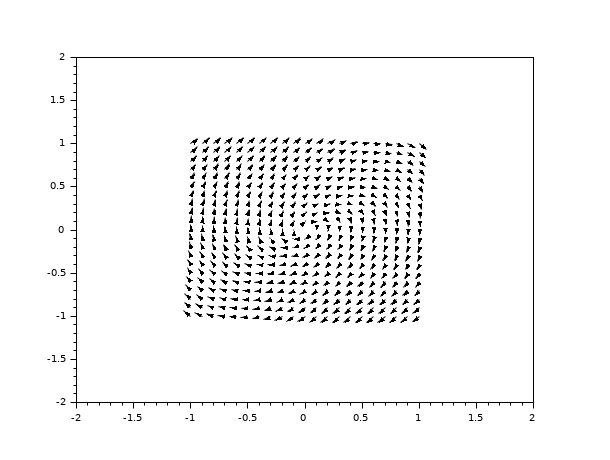Scilab Home page | Wiki | Bug tracker | Forge | Mailing list archives | ATOMS | File exchange
Change language to: Français - Português - 日本語 - Русский
Scilab Help >> Graphics > 2d_plot > fchamp

fchamp

direction field of a 2D first order ODE

Syntax

fchamp(f, t, xr, yr, [arfact, rect, strf])
fchamp(f, t, xr, yr, <opt_args>)

Arguments

f

an external (function or character string) or a list which describes the ODE.

-

It can be a function name f, where f is supposed to be a function of type y=f(t, xy, [p1, ..., pn]). f returns a column vector of size 2, y, which gives the value of the direction field f at point xy=[x,y] and at time t.

-

It can also be an object of type list, list(f,P1, ..., Pn) where f is a function of type y=f(t, xy, p1, ..., pn) and Pi gives the value of the parameter pi.

t

the selected time.

xr, yr

two row vectors of size n1 and n2 which define the grid on which the direction field is computed.

<opt_args>

This represents a sequence of statements key1=value1, key2=value2,... where key1, key2,... can be one of the following: arfact, rect, strf (see below).

arfact, rect, strf

Optional arguments, see champ.

Description

fchamp is used to draw the direction field of a 2D first order ODE defined by the external function f.Note that if the ODE is autonomous, argument t is useless, but it must be given.

Enter the command fchamp() to see a demo.

Examples

deff("[xdot] = derpol(t,x)",..
["xd1 = x(2)";..
"xd2 = -x(1) + (1 - x(1)**2)*x(2)";..
"xdot = [ xd1 ; xd2 ]"])
xf= -1:0.1:1;
yf= -1:0.1:1;
fchamp(derpol,0,xf,yf)deff("[xdot] = derpol(t,x)",..
["xd1 = x(2)";..
"xd2 = -x(1) + (1 - x(1)**2)*x(2)";..
"xdot = [ xd1 ; xd2 ]"])
xf= -1:0.1:1;
yf= -1:0.1:1;
clf()
fchamp(derpol,0,xf,yf,1,[-2,-2,2,2],"011")